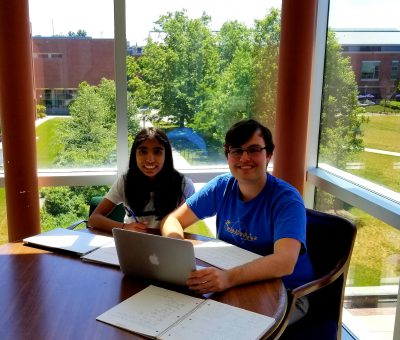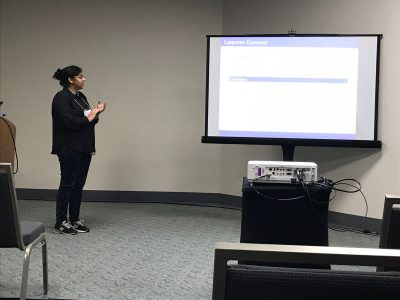# Resistance scaling on the Octacarpet

## Group Members## Overview

The study of analytic structures on self-similar fractal sets was initiated by physicists who discovered that heat flow on such sets had sub-Gaussian rather than Gaussian scaling, indicating that the fundamental physics of these sets was very different than on manifolds. These results were first made rigorous for sets with a finite ramification property, but in the late 1980s, Barlow and Bass developed a corresponding theory on a class of generalized Sierpinski carpets. Their approach depends on taking a (weak) limit of Brownian motions on a suitable sequence of closed sets that intersect to the carpet. A key step in proving that the limiting object has sub-Gaussian scaling is showing that the resistance of the approximating domain of scale n is bounded above and below by ρ^n
for a factor ρ that depends on the carpet. Computing the exact value of ρ remains an open problem.

We consider the resistance scaling problem for the octacarpet, and more generally for 4N-carpets, with
the goal of showing analogous bounds for the resistance and obtaining numerical estimates for the resistance scaling factors.

# Geodesic Interpolation on the Sierpinski Gasket

## Overview

Geodesics (shortest paths) on manifolds such as planes and spheres are well understood.  Geodesics on fractal sets such as the Sierpinski Triangle are much more complicated.  We begin by constructing algorithms for building shortest paths and provide explicit formulas for computing their lengths.  We then turn to the question of interpolation along geodesics—given two subsets of the Sierpinski Triangle, we “slide” points in one set along geodesics to the other set.  We construct a measure along the interpolated sets which formalizes a notion of the interpolation of a distribution of mass, and we prove interesting self-similarity relations about this measure.

# Computations on the Koch Snowflake with Boundary and Interior Energies

## Group Members## Overview

At the end of the 20th century studies had been conducted on the Koch Snowflake which had been motivated through work done by physicists on “fractal drum” experiments. These investigations focused on the eigenfunctions of the negative Dirichlet Lapcian generated on a planar domain with a fractal boundary, particularly with the condition that the boundary be set to zero. Here we study the eigenfunctions on the Koch Snowflake with a non-zero boundary condition and we consider a Laplacian defined on the boundary. To generate an n-level fractal and apply the Laplacian matrix, Python programming was implemented for both the n-level fractal and Laplacian matrix. This then gives us insight into the eigenvalues and visualization of the corresponding eigenfunctions on the Koch Snowflake. Initial observations indicate a kind of localization of the eigenfunctions on the fractal boundary.

# Lipschitz Continuity of Laplacian Eigenfunctions on a class of postcritically finite (PCF) self-similar sets

## Group Members## Overview

We prove a general result for Lipschitz and Hölder continuity of functions defined on a class of postcritically finite (PCF) self-similar sets. Intuition for this theorem comes from formulating arguments on the unit interval, which is well understood in a classical setting. We generalize these results for many kinds of self-similar sets endowed with different measures and metrics. As a corollary to this general result, we prove that eigenfunctions of the Laplacian on the harmonic Sierpinski Gasket are Lipschitz continuous.

# Probability, Analysis and Mathematical Physics on Fractals 2018

Each year we are looking for a group of undergraduate students to work on Probability, Analysis and Mathematical Physics on Fractals. The aim of the projects will be exploration of differential equations and various operators on fractal domains. Students in the project are supposed to have the usual background in linear algebra and differential equations. Knowledge of Matlab, Mathematica, other computer algebra systems, or programming, as well as proof writing, mathematical analysis, and probability may be helpful but is not required. Previous undergraduate work includes published papers on the eigenmodes (vibration modes) of the Laplacian (2nd derivative) of functions that live on Sierpinski gasket type fractals, and the electrical resistance of fractal networks, as well as work on Laplacians on projective limit spaces. The exact choice of the topics to study will depend on the students’ background and interests. Besides being interesting, taking part in a research project like this may be very useful in the future (for instance, when applying to graduate schools).

# Financial Math: Portfolio Optimization and Dynamic Programming

## Group Members

Ayelet Amiran, Skylyn Brock, Ryan Craver, Ugonna Ezeaka, Mary Wishart## Overview

Financial markets have asymmetry of information when it comes to the prices of assets. Some investors have more information about the future prices of assets at some terminal time. However, what is the value of this extra information?

We studied this anticipation in various models of markets in discrete time and found (with proof) the value of this information in general complete and incomplete markets. For special utility functions, which represent a person’s satisfaction, we calculated this information for both binomial (complete) and trinomial (incomplete) models.

## Publication

 Journal reference: Involve 12 (2019) 883-899 DOI: 10.2140/involve.2019.12.883

# arXiv:1808.03186

tba

## Poster

PosterFinMath2018

## Overview

Laplacians have been well studied on post-critically finite (PCF) fractals. However, less is known about gradients on such fractals. Building on work by Teplyaev, we generalize results regarding the existence and continuity of the gradient on the standard Sierpinski Gasket to higher dimensional Sierpinski Gaskets. In particular, we find that, for functions with a continuous Laplacian, the gradient must be defined almost everywhere, and specify a set of points for which it is defined. Furthermore, we provide a counterexample on higher-dimensional Sierpinski gaskets where the Laplacian is continuous but the gradient is not defined everywhere. We conjecture that Hölder continuity of the Laplacian is a condition strong enough to guarantee that the gradient exists at each point.

# Spectral Analysis on Graphs Related to the Basilica Julia Set

## Overview

We analyze the spectra of a sequence of graphs constructed from the Schreier graphs of the Basilica group.  Our analysis differs from earlier work of Grigorchuk and Zuk in that it is based on a macroscopic decomposition of the graphs. This method gives precise information about the multiplicities of eigenvalues and, consequently, good information about the spectral measures of large graphs. It also permits a proof of the existence of gaps in the spectrum of limiting graphs.

# Multiplicative LLN and CLT and their Applications

## Overview

We study the Law of Large Numbers (LLN) and and Central Limit Theorems (CLT) for products of random matrices. The limit of the multiplicative LLN is called the Lyapunov exponent. We perturb the random matrices with a parameter and we look to find the dependence of the the Lyapunov exponent on this parameter. We also study the variance related to the multiplicative CLT. We prove and conjecture asymptotics of various parameter dependent plots.

## Presentations:

Raji Majumdar and Anthony Sisti, will present posters Applications of Multiplicative LLN and CLT for Random Matrices and Black Scholes using the Central Limit Theorem on Friday, January 12 at the MAA Student Poster Session, and give talks on Saturday, January 13 at the AMS Contributed Paper Session on Research in Applied Mathematics by Undergraduate and Post-Baccalaureate Students.# Group Members

Megan Brown, Grace WrightSteven Lemay

## Overview

This project was motivated by the current emphasis on argumentation in the Common Core State Standards for Mathematics. In particular, we focused on tasks that address the third standard for Mathematical Practice that highlights argumentation as an expertise all students should develop. Our data consists of 157 tasks from 40 elementary and high school teachers participating in a professional development program focused on mathematical argumentation. We analyzed how argumentation affects the cognitive demand levels of the tasks, as well as how argumentation detracts or contributes to the mathematical content in the tasks. Our findings show a change in cognitive demand levels when tasks were analyzed with and without the argumentation component. In addition, five different themes emerged from our analysis with regards to the interplay of argumentation and mathematical concepts, some of which were common across elementary and high school tasks.

Poster